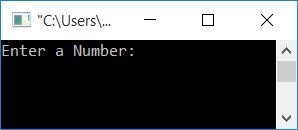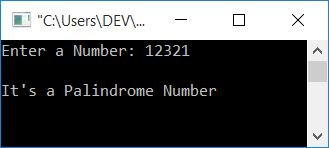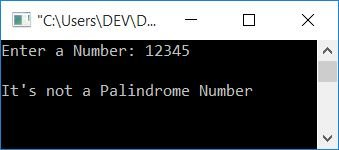# C program to check the palindrome number

In this article, you will learn how to use code to check whether the number given by the user (at run-time) is a palindrome or not. But before going to the program, let's first understand which number can be called a palindrome.

### What is a palindrome number?

If a number is equal to its reverse, it is called a palindrome number. For example, 12321 is a palindrome because its reverse, 12321, is equal to the number itself. Whereas 12345 is not a palindrome number because its reverse (54321) is not equal to the number itself.

## Check if the given number is a palindrome in C

Now let's create a program that checks for a palindrome number.

```#include<stdio.h>
#include<conio.h>
int main()
{
int num, rev=0, rem, temp;
printf("Enter a Number: ");
scanf("%d", &num);
temp = num;
while(temp>0)
{
rem = temp%10;
rev = (rev*10)+rem;
temp = temp/10;
}
if(rev==num)
printf("\nIt's a Palindrome Number");
else
printf("\nIt's not a Palindrome Number");
getch();
return 0;
}```

This program was built and runs in the Code::Blocks IDE. Here is its sample run:Now supply any number, say 12321, as input and press the ENTER key to see the following output:As already explained, if the reverse of a number is equal to the original number, then it will be a palindrome. Therefore, we have just reversed the number and compared it with the original. If it is equal, then print it as a palindrome; otherwise, print it as not a palindrome using the if-else statement.

## Using the for loop, determine the palindrome number

Let us now use a for loop to create the same purpose program.

```#include<stdio.h>
#include<conio.h>
int main()
{
int num, rev=0, rem, temp;
printf("Enter a Number: ");
scanf("%d", &num);
for(temp = num; temp>0; temp=temp/10)
{
rem = temp%10;
rev = (rev*10)+rem;
}
if(rev==num)
printf("\nIt's a Palindrome Number");
else
printf("\nIt's not a Palindrome Number");
getch();
return 0;
}```

It will produce the same output as the previous program. Here is a sample run if the user enters a number whose reverse is not equal to the original one.#### The same program in different languages

C Quiz

« Previous Program Next Program »